# Blog Viewer

### Teaching Fractions with Units Coordination in Mind (Part 2)

#### By Adrianne Burns posted 05-07-2018 07:13

If you found my first post explaining units coordination with whole numbers helpful, this post will give you the details from my experience working with fractions and how impactful it is to students when understanding units coordination.

Fraction problems like this are typical in my 7th-grade classroom:

Is ½ of ⅘ more or less than ⅘?

If ½ pound costs \$3.50, what is the price per pound?

In all fraction problems, there exists one whole of which the fraction is part.  By middle school, most students have the part-whole understanding that ½ is one out of 2 parts of the whole unit.   If a student is struggling with the second question above, a sketch like the one below will help him find the answer.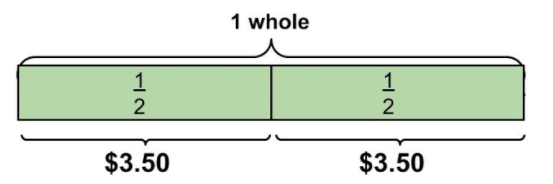But stopping here fell short.  Students were unable to figure out related problems.  What if it were ¾ of a pound? What if I needed the cost for 1 ¼ pounds?

I have found that students are able to calculate with fractions by following memorized rules.  I helped struggling students better understand the rules, leading discussions on why we do or do not need a common denominator, and using manipulatives to show what’s happening as we work with fractions.  By understanding the role of units coordination, my fraction instruction changed. I realized that what students were struggling with was flexibility in fractional thinking.

A seventh-grade student asked me how we could put ½ on a number line because the number line is infinite.  This student--and many like him--only have a part-whole understanding of fractions.

To understand and work with fractions students need to see ½ as a unit of its own - not just part of the whole.

This requires 2 levels of units coordination - understanding the whole as a unit and ½ as a unit within it.

For students to work flexibly with 3 levels of units, they need to see that ¾ is more than 3 out of 4 pieces.  Students need to understand that there is a whole (4/4), a unit fraction (¼), and that ¾ is a unit made up of 3 - ¼ parts.

Thinking back to hierarchical inclusion mentioned in the last post, what does this look like with fractions?  What units are included in a number such as 5/3? Does the student see the unit fraction?  Does the student understand that 1 whole is included in that number?

Just like in the example of two wholes from my first blog post, I had a student shout out in excitement when multiplying fractions, “Oh, I get it.  ⅘ is the new whole!”  When we think of the whole in fractions we typically think about 1.  In order to find ½ of ⅘, we must think of ⅘ as the whole and we want half of it.

If we want students to think flexibly, we need to show them the flexibility of these numbers.

For me, this meant I needed to move my students beyond the part-whole visuals.

One of my favorite tools is Desmos, and when it comes to fraction activities, Kendra Lockman is queen.  In this activity, she connects part-whole thinking with number lines.  All of Kendra’s Desmos activities are posted on her blog as well as Desmos tools she created that can be copied and pasted into your own activities.

Fractions on number lines have been essential to deepening students fraction sense.  A great routine has been the combination of Fraction Talks and Clothesline Math as seen in the tweet below: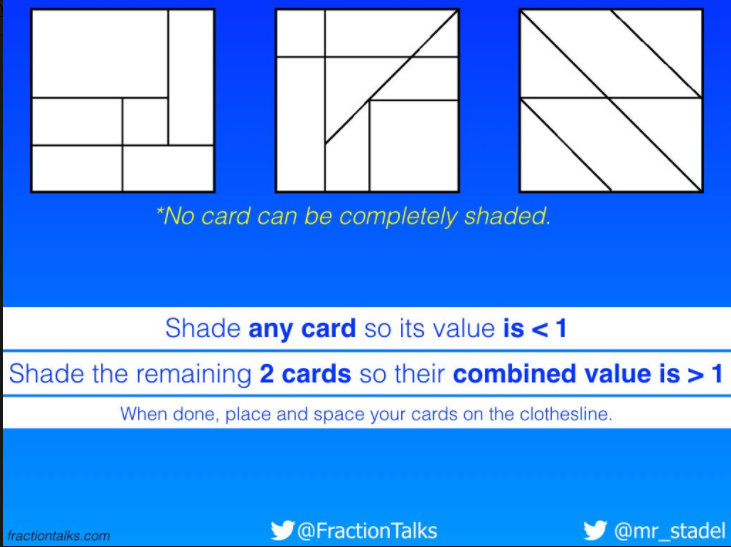For more fraction clothesline ideas, I suggest
Nat Banting’s Clothesline Series and Andrew Stadel’s Clothesline Resources.  Number strings are a sequence of problems and this idea by Graham Fletcher uses a number line in a number string to build students understanding.

Cuisenaire rods are another essential resource. Much like the bars tasks of the units coordination assessment, asking purposeful questions with Cuisenaire rods can get students thinking about the relationships between the different units and moving flexibly between them.  Here is an example: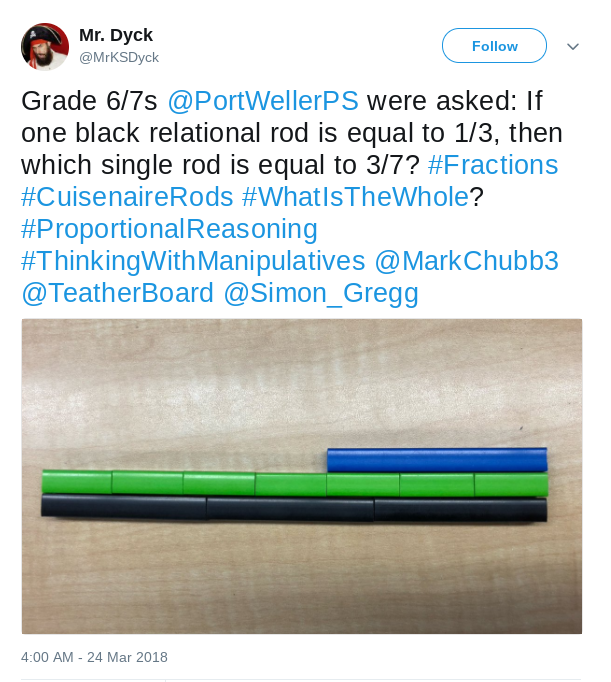I blogged about my work with Cuisenaire rods with fraction division.  If the purple rod is ⅖, which rod is the whole?  Which rod is ⅗? How many times does ⅖ go into ⅗?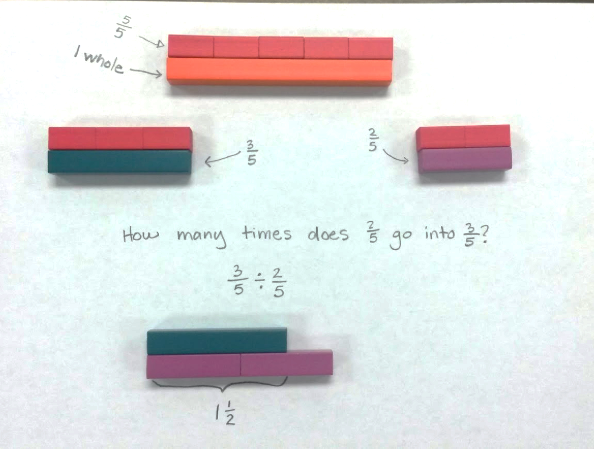If you are curious about your own flexibility with units coordination, I will leave this tweet here as a challenge to you.  It illustrates just how complex fractional thinking can be.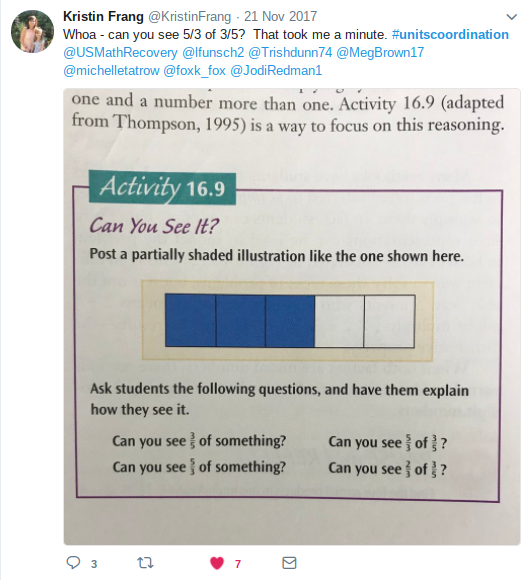(Answers can be found on page 30-31 of this document.)